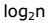# Concept of Binary Search A+ A  A-

If we have ‘n’ records which have been ordered by keys so that x1 < x2 < … < xn . When we are given a element ‘x’, binary search is used to find the corresponding element from the list. In case ‘x’ is present, we have to determine a value ‘j’ such that a[j] = x (successful search). If ‘x’ is not in the list then j is to set to zero (un successful search).

In Binary search we jump into the middle of the file, where we find key a[mid], and compare ‘x’ with a[mid]. If x = a[mid] then the desired record has been found. If x < a[mid] then ‘x’ must be in that portion of the file that precedes a[mid]. Similarly, if a[mid] > x, then further search is only necessary in that part of the file which follows a[mid].

If we use recursive procedure of finding the middle key a[mid] of the un-searched portion of a file, then every un-successful comparison of ‘x’ with a[mid] will eliminate roughly half the un-searched portion from consideration.

Since the array size is roughly halved after each comparison between ‘x’ and a[mid], and since an array of length ‘n’ can be halved only abouttimes before reaching a trivial length, the worst case complexity of Binary search is about.

Time Complexity:

The time complexity of binary search in a successful search is O(log n) and for an unsuccessful search is O(log n).